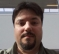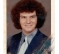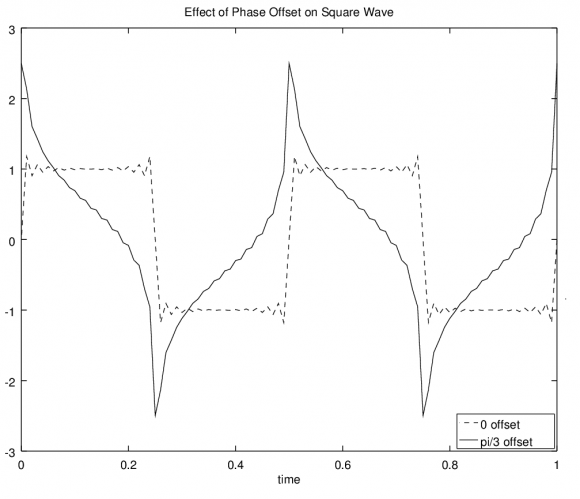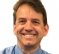# The Most Interesting FIR Filter Equation in the World: Why FIR Filters Can Be Linear Phase

This blog discusses a little-known filter characteristic that enables real- and complex-coefficient tapped-delay line FIR filters to exhibit linear phase behavior. That is, this blog answers the question:

What is the constraint on real- and complex-valued FIR filters
that guarantee linear phase behavior in the frequency domain?

I'll declare two things to convince you to continue reading.

Declaration# 1:
"That the coefficients must be symmetrical" is not a correct

Declaration# 2:
The complex-valued FIR filter coefficients shown in Figure 1(a),
whose positive-frequency magnitude response is shown in Figure 1(b),
exhibit linear phase in the frequency domain. However, interestingly,
neither the real nor the imaginary parts of the coefficients are
symmetrical!Figure 1: Complex-valued FIR coefficients: (a) the
complex coefficients; (b) the coefficients'
frequency magnitude response.

If you would like to know why the asymmetrical coefficients in Figure 1(a) yield a linear-phase FIR filter, please read on.

Real-Valued FIR Filter Coefficients
Let's consider real-coefficient tapped-delay line FIR filters first, such as that shown in Figure 2.Figure 2: N-tap tapped-delay line FIR filter.

The contraint on the $h(n)$ coefficients of a real-coefficient FIR filter guaranteeing linear phase is:

$$\sum_{n=0}^{N-1} h(n) sin [(n - D) \omega] = 0. \tag{1}$$

Equation (1) tells us that if the sum of the products of the FIR filter's N-length $h(n)$ real-valued coefficients times an N-length sine wave sequence, for all $\omega$ in the range of $- \pi \le \omega \le \pi$ radians/sample, is equal to zero then the real-coefficient filter will have a linear phase response. Variable $D$ is the time delay through the filter measured in samples and the integer n index has the range $0 \le n \le N-1$.

The earliest mention of Eq. (1) that I could find in the DSP literature is reference . The Eq. (1) constraint is revisited in reference , and mentioned (without a formal proof) in reference . My derivation of Eq. (1) is given in Appendix A of the downloadable PDF file associated with this blog. (To keep this blog manageable in length, all Appendices are only included in the associated PDF file.)

If you model Eq. (1) in software using various values of $D$ and $\omega$, as I did, you'll find that the only way to satisfy that constraint for an N-tap FIR filter, over the full range of $\omega$, is to: (1) let $D = (N - 1) / 2$ and (2) ensure that your real-valued $h(n)$ coefficients are symmetrical. That is, for real-coefficient FIR filter linear phase,

$$h(n) = h(N-1-n). \tag{2}$$

So this reinforces what we already knew about FIR filters having real-valued coefficients. However, Eq. (1) does not help us explain how the complex-valued coefficients in Figure 1(a) yield a linear-phase filter.

Complex-Valued FIR Filter Coefficients
In trying to learn why the asymmetrical complex coefficients in Figure 1(a) produce a linear-phase filter I generated an expression similar to Eq. 1. For an N-tap FIR filter having complex coefficients in the form of $h(n) = h_I(n) + jh_Q(n)$ my derivation, given in Appendix B of the downloadable PDF file associated with this blog, produced the following Eq. (3).

$$\sum_{n=0}^{N-1} h_Q(n) cos [(n-D) \omega + \beta] = \sum_{n=0}^{N-1} h_I(n) sin[(n-D) \omega + \beta] \tag{3}$$

So, Eq. (3) is the constraint on the coefficients that must be satisfied for an N-tap complex-coefficient FIR filter to have linear phase.

Sadly, while studying Eq. (3), my limited analysis skills did not enable me to find an easier to understand expression for the constraint on the coefficients that must be satisfied for an N-tap complex-coefficient FIR filter to have linear phase. I wanted an expression that was as easy to understand as Eq. (2)! Fortunately there is a way to obtain the expression I wanted.

A More Understandable Complex FIR Filter Constraint Expression
The derivations of Eqs. (1) and (3) used the forward discrete time Fourier transform (DTFT). If we, instead, use the inverse DTFT we can obtain an easy to understand expression for the constraint on an FIR filter's complex coefficients. (For a detailed description of the forward and inverse DTFT, see reference .)

Using the inverse DTFT, as was done in my derivation in Appendix C of the downloadable PDF file associated with this blog, the simple expression I obtained is:

$$h(n) = e^{j2\beta}h^*(N-1-n) \tag{4}$$

where $\beta$ is the phase of the complex filter at $\omega = 0$ radians (zero Hz), $N$ is the number of filter taps, index variable $n$ satisfies $0 \le n \le N-1$, and the '$*$' symbol means complex conjugate.

The easy to understand Eq. (4) is the little-known constraint on the coefficients that must be satisfied for an N-tap complex-coefficient FIR filter to have linear phase! And happily, Eq. (4) also applies to real-coefficient FIR filters because in that case, since $\beta = 0$, Eq. (4) simplifies to our above Eq. (2). And, of course, Eq (4) is valid for both odd and even values of $N$.

Due to the popularity of complex FIR filtering in recent years, I believe Eq. (4) should be included in every new DSP textbook. Let the record show that I now formally nominate Eq. (4) as 'The Most Interesting FIR Filter Equation in the World'.A Matlab Example
For those readers who find this topic interesting, and have Matlab software, I supplied the Matlab code I used to generate the $h(n)$ coefficients in Figure 1(a) and verify the properties of Eq. (4) in the downloadable PDF file associated with this blog. Executing that Matlab code gives us the following complex values for Figure 1(a)'s nine $h(n)$ complex-valued coefficients:

h(0) = -0.0592
h(1) = 0.1132 + 0.0822i
h(2) = 0.0562 + 0.1729i
h(3) = -0.0721 + 0.2218i
h(4) = -0.2061 + 0.1497i
h(5) = -0.2332 + 0.0000i
h(6) = -0.1471 - 0.1069i
h(7) = -0.0432 - 0.1330i
h(8) = -0.0183 + 0.0563i

For Further Study
The next thing I need to figure out is: When the complex-coefficients are quantized what effect does that have on a complex FIR filter's phase linearity.References

 L. Rabiner and B. Gold, "The Theory and Application of Digital Signal Processing", Prentice Hall, Englewood Cliffs, New Jersey, 1975, pp. 78-79.

 A. Oppenheim, R. Schafer, and J. Buck, "Discrete-Time Signal Processing", 2nd Ed., Prentice Hall, Englewood Cliffs, New Jersey, 1999, Section 5.7.3, pp. 296-297.

 Wikipedia: "Linear Phase", https://en.wikipedia.org/wiki/Linear_phase

 Sabanci University, Istanbul, Turkey, 2004, http://abut.sdsu.edu/TE302/Chap4.pdf

[ - ]
Comment by May 1, 2016Have you analyzed the affects of quantization?
I'm about to design a decimation filter for software-defined radio and I wanted to compare the performance of complex vs. real valued filters.
A filter with complex coef. (as opposed to real) will require more computation but I am hoping this can be offset by an improvement in performance (require fewer taps).
[ - ]
Comment by May 1, 2016Hello Quazil. Ah, such a good question. No, I did not experiment with the behavior of my Equation (4) when beta and h(n) were quantized. It's been my experience when working with complex-valued filters that having quantized coefficients disrupts (prevents), to some degree, the desired 90-degree phase relationship between various sequences that exist in complex filtering applications. Your question makes me wonder, "In my Eq. (4), what is the effect of having beta and h(n) quantized to 16 bits." I'll experiment with this question and post anything interesting that I learn. Quazil, when you start designing your decimation filters, if you have problems you can post questions on the dsp.related.com forum for help. The forum is at:

https://www.dsprelated.com/forums
[ - ]
Comment by August 19, 2015It has been a long while since I have learned about the linear phase property of a FIR filter. I ended up spending a couple hours reading Oppenheim & Schafer DSP book to refresh my memory. I would like to thank the author for the constraint for complex FIR filter. This is new to me and actually this is not mentioned in Oppenheim & Schafer DSP book. Yes, I agree that the complex-valued constraints should be added to our DSP text book. I also have the following comments.

Comment 1
----------------
For the case where the FIR filter has real-valued cofficients, I think there are 4 constraints (the blog article only mentions 2 of the 4 constraints) on the FIR filter cofficients that will guarantee linear phase response.

Type 1: h[n] = h[M - n] for 0 <= n <= M and M is even. This is the same as equation (2) in the blog article.

Type 2: h[n] = h[M - n] for 0 <= n <= M and M is odd. This is the same as equation (2) in the blog article.

Type 3: h[n] = -h[M - n] for 0 <= n <= M and M is even.

Type 4: h[n] = -h[M - n] for 0 <= n <= M and M is odd.

Comment 2
----------------
In page 2 of the blog article, we can find the following sentence,

So, Eq. (3) is the constraint on the coefficients that must be satisfied
for an N-tap complex-coefficient FIR filter to have linear phase.

I am not sure about the phrase "must be satisified". I think all the constraints that we can come up with are only sufficient conditions. These contstaints are not necessary conditions. Therefore it is possible to find a FIR filter (whether complex or real) having a linear phase frequency response but does not satisfy any constraint that we mention. I am guessing (not verify yet) that one way to create such a FIR filter is to take a FIR filter (that satisfies our constraint) and then do a fractional delay shift of this FIR filter impulse response. Just my guess.
[ - ]
Comment by August 21, 2015Hello cykhung,
Thanks for your thoughts. Regarding your Comment 1, I purposely omitted your Type 3 and Type 4 constraints from my blog. Those constraints apply to digital differentiators and Hilbert transformers but they don't apply to what we traditionally call "FIR filters."

Regarding your Comment 2, you wrote:

"Therefore it is possible to find a FIR filter (whether
complex or real) having a linear phase frequency response
but does not satisfy any constraint that we mention."

Oppenheim's & Schafer's 3rd edition does mention the notion of lowpass filter having linear phase but whose real-valued impulse response is NOT symmetrical. However, that filter's impulse response must be infinite in length (infinite time-duration), and therefore is not an FIR filter.
[ - ]
Comment by August 26, 2015Regarding comment 2, is the following statement correct?

All complex-valued FIR filter having constant group delay must satisfy Equation 4.
[ - ]
Comment by August 26, 2015Hello cykhung,
I developed a proof showing that all complex-valued FIR filters that satisfy Equation 4 will have constant group delay. (I chose not to include that proof in the PDF file associated with this blog.)

[ - ]
Comment by August 26, 2015Hi Rick,

Thanks for the reply. I understand what you mean. But I think you and I are saying something slightly different. What you are saying is that:

If a complex-valued FIR filter satisfy Equation 4 ===> then this complex-valued FIR filter will have constant group delay

So I agree with that. However, the reverse may not be true, i.e. is the following true?

If a complex-valued FIR filter has constant group delay ===> then the complex-valued FIR filter must satisfy Equation 4

Thanks
Kevin
[ - ]
Comment by August 27, 2015Hello cykhung,
I cannot say that: all tapped-delay line (convolutional) FIR filters having linear phase must satisfy Equation 4. cykhung, if you know of a linear phase tapped-delay line FIR filter that does not satisfy Equation 4 I am very interested in learning about it.
[ - ]
Comment by August 27, 2015Game On. Let start the search !!!!
[ - ]
Comment by September 20, 2015A very good article from Rick as always. I think it was very smart to allow the phase response of the FIR filter at zero frequency to be non-zero. It is that constraint which really forces the filter coefficients to be symmetric.
[ - ]
Comment by September 22, 2016Hi Rick,

Nice article so far! I'm not done reading it yet.

I was very interested to see your derivation of equation 1 and so had downloaded the PDF which has that derivation in Appendix A and I see a small error where you state, "To help make you comfortable with Eq. (A-5), both sides of that equation are equal to tan(dw)." Well, tan = sin/cos, not cos/sin.
[ - ]
Comment by September 22, 2016Hi Randy,
Ah, you are correct. I inadvertently inverted my Eq. (A-5). Nice catch Randy. I will send a corrected PDF file to the web master Stephane Boucher.
[ - ]
Comment by October 15, 2016Hi Rick,

First I want to say that I liked your book ;"Understanding Digital Signal Processing" so much that I used it to teach DSP to practicing engineers. Good job!

Comment 1 - I think your equation 4) would yield more insight if you multiplied each side by exp(-jB). If you then express the filter coefficients in polar form (rather than the usual rectangular) you can see that these rotated coefficients (rotated by -B) are complex conjugates.

Comment 2 - While I agree that that multiplying the coefficients by the exp(-jB) term allows for constant group delay, it does not give constant time delay except when B=0. (Time delay is phase/frequency.) The only reason I have used linear phase filters is to keep all the frequency components time aligned. Is there any other reason that I am missing?

Comment 3 - With regard to quantized coefficients: As noted in comment 1, the coefficients are complex conjugates so that even with quantized coefficients there is no phase error. We would use the same table value for each of the conjugate terms. Of course, the magnitude response of the filter would be affected by the coefficient errors.

Thanks for the insightful blog.

[ - ]
Comment by October 16, 2016Hello John_G,

Thanks for your kind words regarding my "Understanding DSP" book."I appreciate them.

Regarding your 'Comment 1': Yes, you are correct. The sequence h(n)exp(-jBeta) is conjugate symmetric.

Regarding your 'Comment 2': I view the notion of group delay to be equivalent to "time delay". I say that because the dimension of a group delay value is time measured in seconds. Sometimes people measure group delay in "samples", but in that case a "sample" is an interval in time equal the reciprocal of the Fs sample rate measured in seconds. John_G, if you and I have a disagreement here I'll bet it's a semantics problem rather than a disagreement regarding fundamental signal theory.

Regarding your 'Comment 3': The h(n) coefficients in my Equation (4) do not exhibit any sort of conjugate symmetry. In my software modeling no 'real or 'imaginary' numerical value of any single coefficient is equal to the 'real or 'imaginary' numerical value of any other coefficient.

John_G, if you'd like the errata to your copy of my "Understanding DSP" book" you're welcome to send me a private e-mail at: R_dot_Lyons_at_ieee_dot_org.

[ - ]
Comment by October 18, 2016Hi Rick,

Perhaps I should start by saying that I have spent much of my career (now retired) involved with analog and digital communication where group delay and time delay are very important. I hope I can demonstrate that they are not the same thing.

Wikipedia calls time delay ‘phase delay’ and defines them as follows;

Additionally, it can be shown that the group delay,, and phase delay,, are frequency-dependent, and they can be computed from the phase shiftby.

So for "linear" phase = -aw-b, the group delay = a and phase delay = a + b/w.

Now for narrow-band bandpass filters, b/w is insignificant and so phase and group delay are the same. However, for low pass filters the b/w term blows up as w->0 and so the time delay is not constant.

To demonstrate this distortion I wrote an Octave program simulating the effect of low pass filtering a digital stream with b=0 and b=pi/3. As you can see there is severe distortion when b=pi/3 that would increase the BER.So this is why I question why anyone would want a linear phase filter whose phase does not go through the origin.

Best Regards,

Appendix - Octave Program

% Simple Demonstration of Effect of Phase Offset on Pulse Shape
% Pass Square Wave through Low-Pass Linear Phase Filter
% Filter passes up to 25th harmonic with linear phase
% Filter #1 has constant time delay (linear phase with zero  phase at 0Hz)
% Filter #2 has constant group delay (linear phase with Phase  Offset of pi/3)
y1=0;                       %Initialize Output Waveforms
y2=0;
w=2*pi*2;                   %Frequency = 2 Hz
t = [0:.01:1];              %Time
for n=1:2:25;               %Harmonic Index
y1 = y1+4/pi/n*sin(n*w*t+0);
y2 = y2+4/pi/n*sin(n*w*t+pi/3);
endfor;
%Plot Results
close all;
figure;
hold on;
plot(t,y1,'--k;0 offset;');
plot(t,y2,'-k;pi/3 offset;');
legend('location','southeast');
xlabel('time')
title('Effect of Phase Offset on Square Wave')
hold off;

[ - ]
Comment by October 20, 2016Looking at your two waveforms, you generated a signal that's the sum of odd harmonics (with decreasing peak amplitudes as the frequency is increased), each with an initial phase of zero radians to generate a square-wave. Then you generated a signal that's the sum of odd harmonics (with decreasing peak amplitudes as the frequency is increased), each with an initial phase of $\pi/2$ radians to generate an "oddball-wave."

I'm not sure what to make of those two waveforms. Should I interpret the square-wave to be the input to a system and the oddball-wave to be the system's output? I'm not sure what that oddball-wave is telling me.

(I consider your two waveforms to be wideband signals. They both have nonzero high-frequency content. And I've always been under the impression that 'phase delay' and 'group delay' have little-to-no physical meanings for a system that passes wideband signals from its input to its output.)

You wrote, " I question why anyone would want a linear phase filter whose phase does not go through the origin." I don't think anyone sits down and decides to design a linear-phase filter whose phase does not go through the origin. The point of my blog was to answer a question that had been "bugging" me for years, that is, "How can a complex-coefficient FIR filter have linear phase when neither the real or imaginary parts of its coefficients are symmetrical?" What I learned was that any complex-valued $h(n)$ set of coefficients that satisfy my above Eq. (4) will have linear phase. And those complex-coefficient FIR filters (at least all the ones I've encountered) have a phase response that is not equal to zero at zero Hz.
[ - ]
Comment by May 29, 2021Nice write-up Rick!  Second paragraph below for you, and first paragraph which is obvious to you may be of interest possibly to other readers: To see it intuitively consider that complex conjugate symmetric (non-causal) coefficients in the time domain will always be real in frequency. The intuition part of it comes from plotting the positive time axis and negative time axis pairs on the complex plane and considering how the sum will be real (throwing intuition away it is a basic FT property that complex conjugate symmetric will be real and antisymmetric will be imaginary in the other domain). For any such result we can delay these non-causal coefficients in time to make it causal, which then only makes the all real frequency response linear phase (a delay in time just adds a linear phase in frequency- which to note is a rotation in frequency).  We can then offset the entire thing in time by a fixed phase which just causes the DC bin to shift in phase by the same amount (but notably this does not effect any other frequency bin).

That said I think the prior comment by John G may have missed that (or I missed something so very interested in your review/comment). He had concluded that your phase offset in time would result in a linear phase in frequency with its own phase offset as given by aw-b and then went from there to show all the problems with that. Given what I said, and we could mathematically further detail by taking the FT of e^j(theta) as being e^j(theta)dirac(omega), then the only thing to be modified in the frequency domain would be DC.  (Assuming your phase offset is a constant offset (rotation) to all coefficients, which is how I read your formula).

To post reply to a comment, click on the 'reply' button attached to each comment. To post a new comment (not a reply to a comment) check out the 'Write a Comment' tab at the top of the comments.